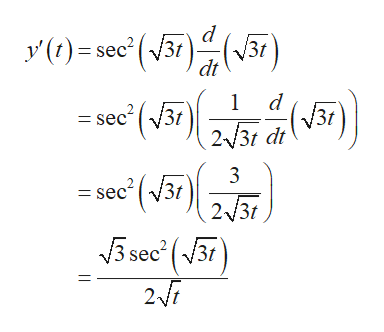# Find the differential of each function.(a)    y=tan(sqrt3t)dy =    (b)    y = 5-v^2/5+v^2dy =

Question

Find the differential of each function.

(a)    y=tan(sqrt3t)

dy =

(b)
y = 5-v^2/5+v^2

dy =

check_circle

Step 1

(a) Find the derivative of the function

Step 2

Differentiate y with respect...help_outlineImage TranscriptioncloseV3r y()=sec(3r) /3t dt d 1 (V31 sec23t /3t 2 3t dt 3 =sec31 2/3t sec(3t (vB fullscreen

### Want to see the full answer?

See Solution

#### Want to see this answer and more?

Solutions are written by subject experts who are available 24/7. Questions are typically answered within 1 hour.*

See Solution
*Response times may vary by subject and question.
Tagged in

### Derivative